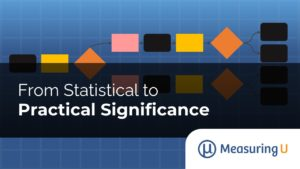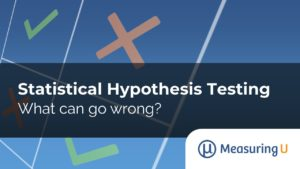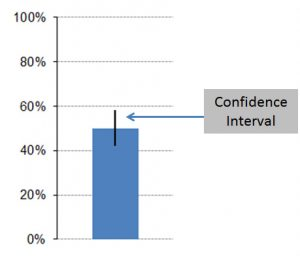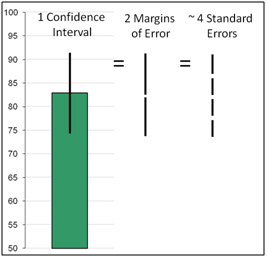### From Statistical to Practical Significance

Hypothesis testing is one of the most common frameworks for making decisions with data in both scientific and industrial contexts. But this statistical framework, formally called Null Hypothesis Statistics Testing (NHST), can be confusing (and controversial). In an earlier article, we showed how to use the core framework of statistical hypothesis testing: you start with### Statistical Hypothesis Testing: What Can Go Wrong?

Making decisions with data inevitably means working with statistics and one of its most common frameworks: Null Hypothesis Significance Testing (NHST). Hypothesis testing can be confusing (and controversial), so in an earlier article we introduced the core framework of statistical hypothesis testing in four steps: Define the null hypothesis (H0). This is the hypothesis that### How Confident Do You Need to be in Your Research?

Every estimate we make from a sample of customer data contains error. Confidence intervals tell us how much faith we can have in our estimates. Confidence intervals quantify the most likely range for the unknown value we’re estimating. For example, if we observe 27 out of 30 users (90%) completing a task, we can be### How to Compute a Confidence Interval in 5 Easy Steps

Confidence intervals are your frenemies. They are one of the most useful statistical techniques you can apply to customer data. At the same time they can be perplexing and cumbersome. But confidence intervals provide an essential understanding of how much faith we can have in our sample estimates, from any sample size, from 2 to### 10 Things to know about Confidence Intervals

You don’t need a PhD in statistics to understand and use confidence intervals. Because we almost always sample a fraction of the users from a larger population, there is uncertainty in our estimates. Confidence intervals are an excellent way of understanding the role of sampling error in the averages and percentages that are ubiquitous in